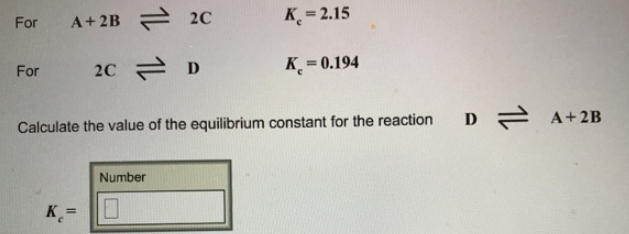# Problem: For A + 2B ⇌ 2C                Kc = 2.15 For 2C ⇌ D                                             Kc = 0.194 Calculate the value of the equilibrium constant for the reaction D ⇌ A + 2B

###### FREE Expert Solution
92% (314 ratings)###### Problem Details

For A + 2B ⇌ 2C                Kc = 2.15

For 2C ⇌ D                                             K= 0.194

Calculate the value of the equilibrium constant for the reaction D ⇌ A + 2B